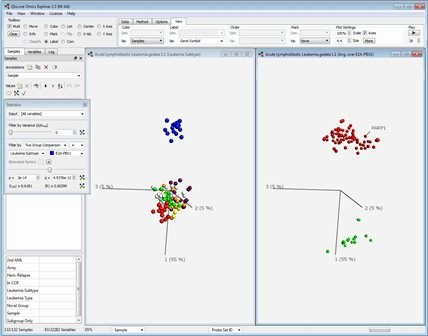# Understanding Principal Component Analysis

PCA is a good technique to reduce the high dimensionality of big data sets to fewer dimensions that are easier for humans to comprehend and visualize.

The very high dimensional nature of many data sets makes direct visualization impossible as we humans can only comprehend three dimensions. The solution is to work with data dimension reduction techniques.

When reducing the dimensions of data, it's important not to lose more information than is necessary. The variation in a data set can be seen as representing the information that we would like to keep.

Principal Component Analysis (PCA) is a well-established mathematical technique for reducing the dimensionality of data, while keeping as much variation as possible.

PCA achieves dimension reduction by creating new, artificial variables called principal components. Each principal component is a linear combination of the observed variables.

PCA is an unsupervised method, meaning that no information about groups is used in the dimension reduction. This means that PCA shows a visual representation of the dominant patterns in a data set.

By calculating, for example, the first three principal components, and visualizing the samples in this three-dimensional space, we can create a visualization containing more of the variance in the original data than any other trio of linear combinations, so in this sense PCA provides the optimal three-dimensional sample representation.

One of the keys behind the success of PCA is that in addition to the low-dimensional sample representation, it provides a synchronized low-dimensional representation of the variables. The synchronized sample and variable representations provide a way to visually find variables that are characteristic of a group of samples. The figure below shows one example of how it might look:Qlucore Omics Explorer, a next-generation bioinformatics software program, can be used to analyse and explore data sets such as:

• Gene expression: microarrays, RNA-seq, real-time PCR
• MicroRNA: microarrays, RNA-seq, real-time PCR
• DNA methylation: microarrays
• Protein expression: microarrays, antibody arrays, 2-D gels, LC-MS
• Any multivariate data of size up to 1500 x 50,000 or 150 x 500,000